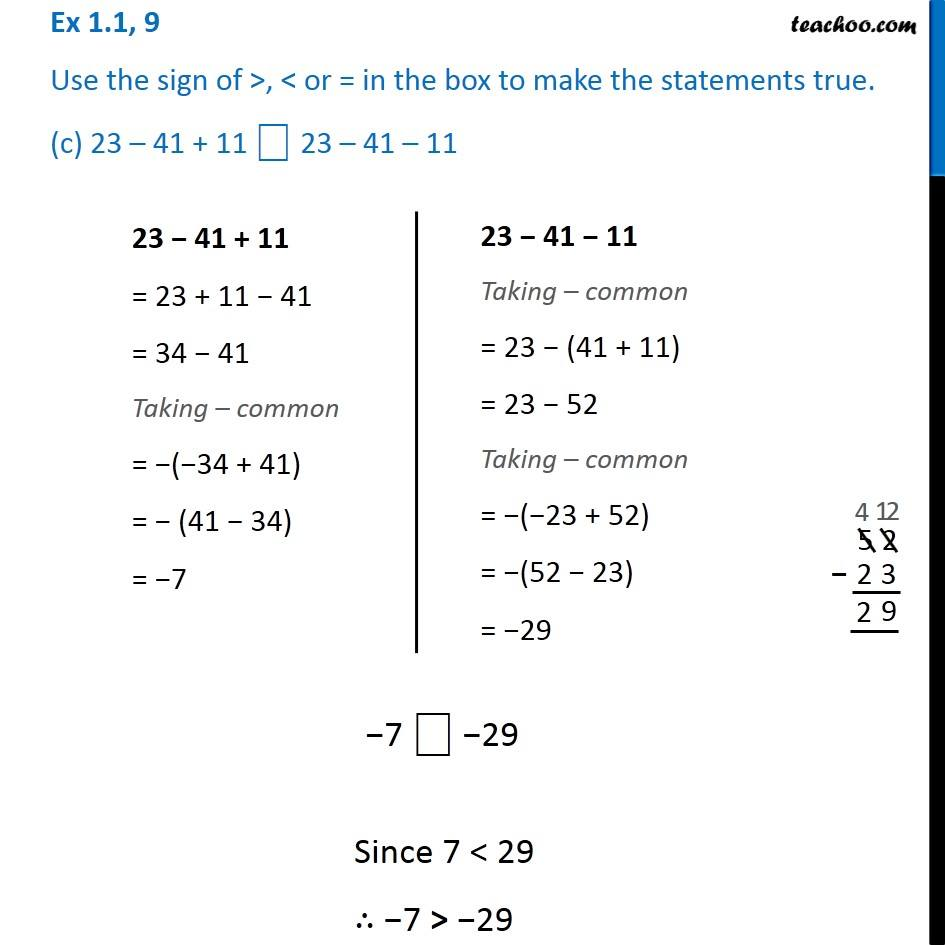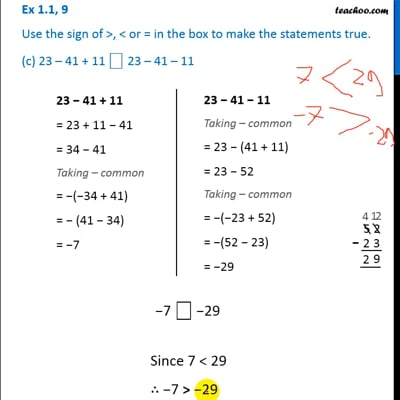Ex 1.1

Chapter 1 Class 7 Integers
Serial order wiseThis video is only available for Teachoo black users

Introducing your new favourite teacher - Teachoo Black, at only ₹83 per month

### Transcript

Ex 1.1, 9 Use the sign of >, < or = in the box to make the statements true. (c) 23 – 41 + 11 ⎕ 23 – 41 – 1123 − 41 + 11 = 23 + 11 − 41 = 34 − 41 Taking – common = −(−34 + 41) = − (41 − 34) = −7 23 − 41 − 11 Taking – common = 23 − (41 + 11) = 23 − 52 Taking – common = −(−23 + 52) = −(52 − 23) = −29 7 ⎕ −29 Since 7 < 29 ∴ −7 > −29